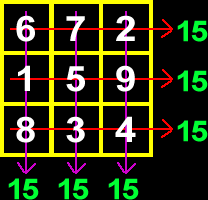# Online Math Dictionary: M

Easy to understand math definitions for K-Algebra mathematics
Just scroll down or click on the word you want and I'll scroll down for you!

 magic square mean median midpoint formula milligram millimeter millisecond minuend Mobius strip mode monomial multiple multiplicity

 Magic SquareA magic square is filled with the numbers 1-9 (each used once) so that the sum of every rowand every column is the same (15 in my example).Mean
The mean of a group of numbers is the same thing as the average.  It's weird to define "average" in words, but really easy  to show what it is.
Look at this list of numbers:  3, 6, 13, 4, 9...   Add them up and we get 35...  Now, divide by how many numbers there are.  There are five numbers, so divide 35 by 5...   We get 7 and that's the average of this list of numbers.  Notice that some of the numbers are above 7 and some are below.MedianThe median is the middle number of a group.    Example:  Here's a list of numbers:            2, 8, 5, 9, 12, 11, 1 Arrange them in order:            1, 2, 5, 8, 9, 11, 12 Count in from the front and the back to find the one that's in the middle... The median is 8.